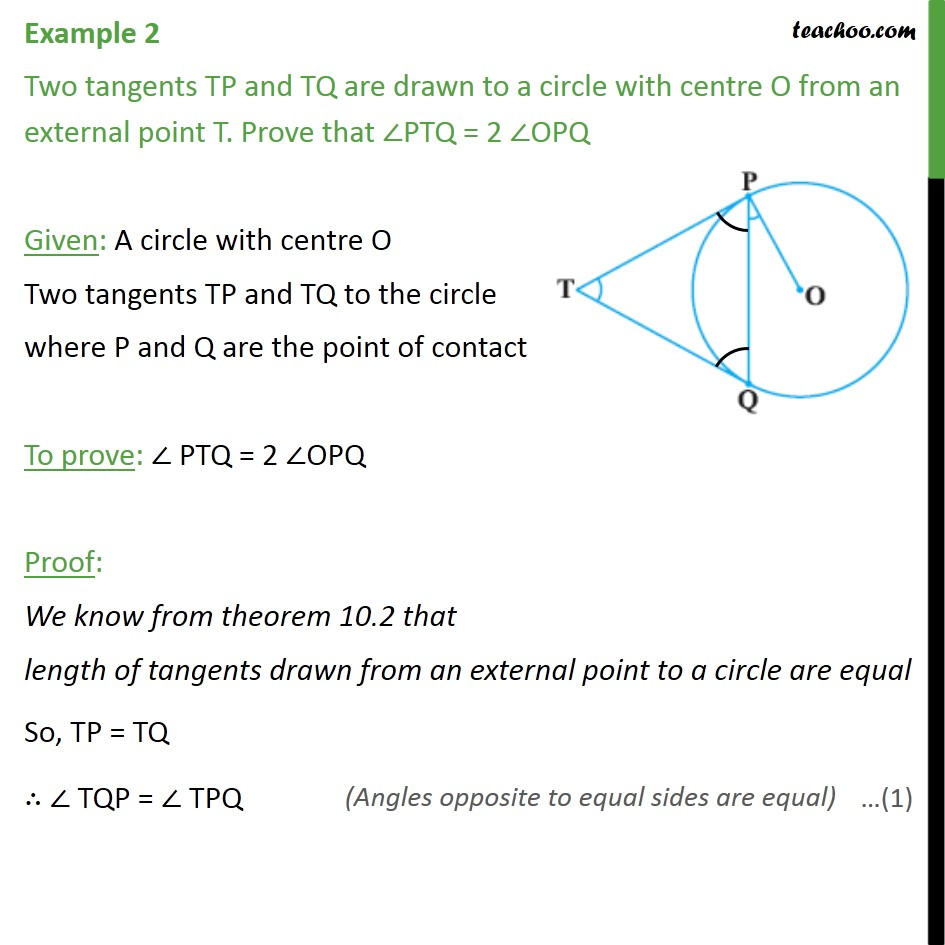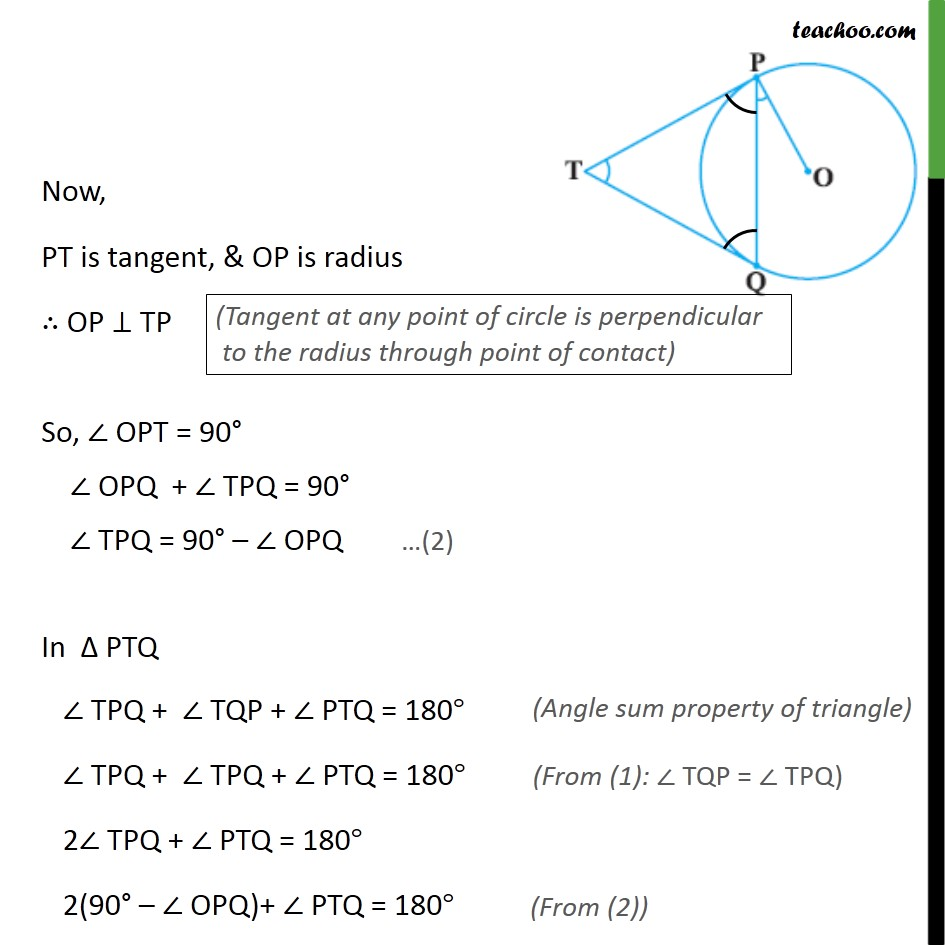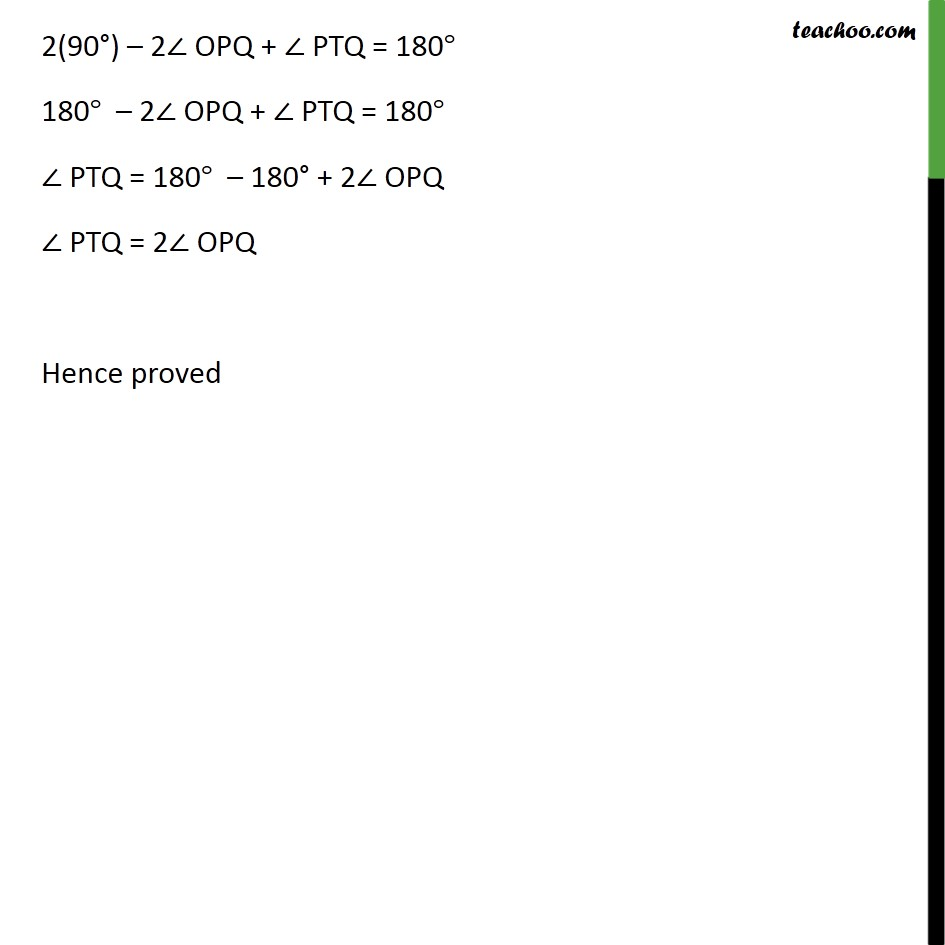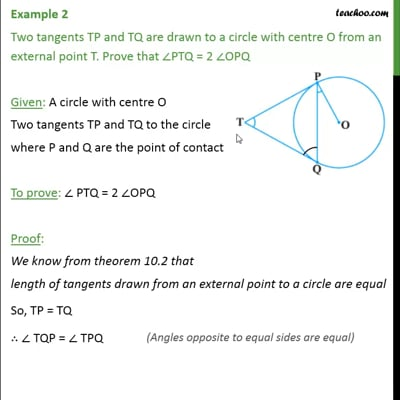Examples

Chapter 10 Class 10 Circles
Serial order wiseThis video is only available for Teachoo black users

Solve all your doubts with Teachoo Black (new monthly pack available now!)

### Transcript

Example 2 Two tangents TP and TQ are drawn to a circle with centre O from an external point T. Prove that ∠PTQ = 2 ∠OPQ Given: A circle with centre O Two tangents TP and TQ to the circle where P and Q are the point of contact To prove: ∠ PTQ = 2 ∠OPQ Proof: We know from theorem 10.2 that length of tangents drawn from an external point to a circle are equal So, TP = TQ ∴ ∠ TQP = ∠ TPQ Now, PT is tangent, & OP is radius ∴ OP ⊥ TP So, ∠ OPT = 90° ∠ OPQ + ∠ TPQ = 90° ∠ TPQ = 90° – ∠ OPQ In Δ PTQ ∠ TPQ + ∠ TQP + ∠ PTQ = 180° ∠ TPQ + ∠ TPQ + ∠ PTQ = 180° 2∠ TPQ + ∠ PTQ = 180° 2(90° – ∠ OPQ)+ ∠ PTQ = 180° 2(90°) – 2∠ OPQ + ∠ PTQ = 180° 180° – 2∠ OPQ + ∠ PTQ = 180° ∠ PTQ = 180° – 180° + 2∠ OPQ ∠ PTQ = 2∠ OPQ Hence proved